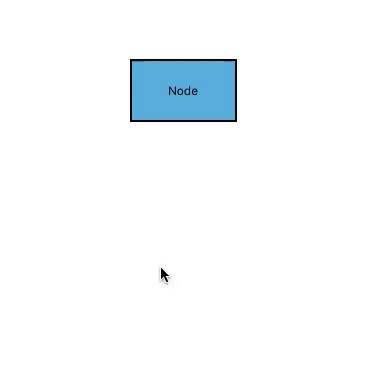# Drawing Mode in Xamarin Diagram (SfDiagram)

Drawing mode is used to draw continuously on the diagram area for selected mode dynamically.

## Text node

This node has default annotation. TextNode mode will add continuous text node. The following code example illustrates how to enable TextNode mode.

``````<diagram:SfDiagram x:Name="diagram" DrawingMode="TextNode">
</diagram:SfDiagram>``````
``diagram.DrawingMode = DrawingMode.TextNode;``

## Connector

Connector mode add continuous orthogonal connectors on the diagram area. In this mode, you can connect connectors in between Points, Nodes, or Ports. The following code example illustrates how to enable Connector mode.

``````<diagram:SfDiagram x:Name="diagram" DrawingMode="Connector">
</diagram:SfDiagram>``````
``diagram.DrawingMode = DrawingMode.Connector;``